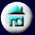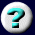11. Conservation of Mass,       Charge, and Energy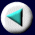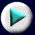Mass and Energy: Nuclear Reactions
 In the fusion reaction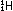and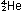should be understood as representing nuclei, with total mass numbers given by superscripts, and nuclear charge (equal to the number of protons) given by subscripts. An electron is represented as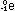, with a zero mass number (not counted among the nucleons) and a -1 charge. In the fusion equation four hydrogen nuclei (not atoms) combine with two of the four electrons around the atoms to form a helium nucleus. The two remaining original electrons, not shown in the equation, associate with the nucleus to build a neutral helium atom. A proton in this representation isp, and a neutron is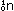. The uranium fission reaction tells us that a uranium nucleus, when bombarded by a neutron, breaks down into barium and krypton nuclei with the release of three more neutrons. Therefore this is a chain reaction, with more neutrons released than were used up. These neutrons can bombard neighboring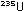nuclei and produce even more fission. Mass number (superscripts) and charge (subscripts) are conserved in nuclear reactions of this type, just as the number of each kind of atom is conserved in a chemical reaction. You should verify that the sum of subscripts, and sum of superscripts, are constant on the two sides of the equation.
Page 27 of 37